# AP Physics B : Using the Lens Equation

## Example Questions

### Example Question #1 : Using The Lens Equation

An object is placed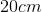in front of a convex thin lens with focal length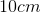. How far is the image from the lens, and is it real or virtual?, virtual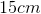, real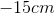, real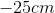, virtual, real, real

Explanation:

For the thin lens equation, the convention for image distance is positive on the opposite side of the lens from the object. In effectively all practical cases for a single thin lens, positive image distance corresponds to a real image, and negative to a virtual. Convex lenses effectively only produce real images for objects outside their focal distance. Based on these initial observations, certain answer choices can be eliminated.

Use the thin lens equation to choose between the remaining answer options.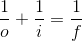In the equation,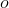is the object distance, oris the focal length, or; andis the image distance from the lens.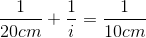Since the object distance is twice the focal length, the image distance will be as well.  So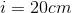, and the image is real, which can be confirmed with a quick ray diagram

.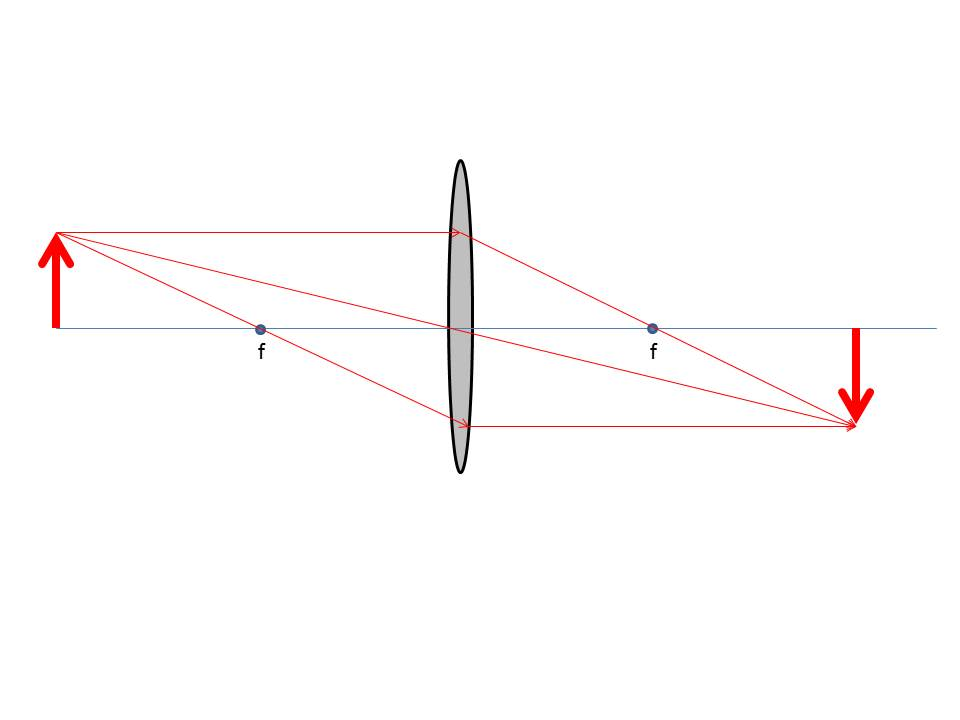The ray diagram for a simple thin lens can be constructed according to three rules that apply, in their own way, either to converging or diverging lenses (convex or concave):

1. A ray traveling parallel to the optical axis gets bent by the lens so as to pass, or appear to pass, through the focal point.
1. A ray traveling through the center of the lens is not deviated.
1. A ray passing through, or appearing to pass through, the focal point of the lens is bent parallel to the optical axis.

Following these rules, it can be seen that the convex lens brings the light rays to a real focus at the image position, and the image would fall on a screen placed at that point to create a real image.

Note:  This configuration represents what is called “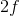to” imaging, where the object is twice as far from the convex lens as its focal length, and thus the image is as well. It turns out that, for a simple convex lens, this is the smallest total object-to-image distance attainable for a real image.